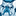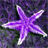QlikView App Dev

Discussion Board for collaboration related to QlikView App Development.

Announcements
QlikWorld 2022, LIVE in Denver CO., May 16-19, 2022. REGISTER NOW TO RECEIVE EARLY BIRD PRICING
cancel
Showing results for
Did you mean:Creator II

variable error in expression

Hi,

Please help me figure out what is wrong with my variable in this expression. It just returns null.

=count({<[Decision Date]={\$(vYesterdayApp)}>}[Decision Result])

This is my variable:

vYesterdayApp = max([Decision Date])

When I create a text obect with just the variable value I get 2013/01/07, which is correct.

19 RepliesMaster II

try this

count({\$<[Decision Date]={\$(#=only(vYesterdayApp))}>}[Decision Result])

hope it helpsPartner

You could ditch the vYesterdayApp variable with:

=count({<[Decision Date] = {"=\$(=Date(Max([Decision Date])))"}>} [Decision Result])

Regards

Jonathan

Logic will get you from a to b. Imagination will take you everywhere. - A EinsteinPartner

vYesterdayApp = max([Decision Date])

when evaluating the expression it is getting the max for each row,Partner

Hi:

Have you tried groupping?

vYesterdayApp = aggr(     max([Decision Date])     , [Decision Result])

It should give you the max for each [Decision Result].Creator II
Author

Tried all 3 above suggestions with no luck.

I like the idea of not using the variable, and rather using max() in my set analysis - but please help me with the syntax.

=count({<[Decision Date] = {"=\$(=Date(Max([Decision Date])))"}>} [Decision Result])

returns a count of all apps.Creator II
Author

Hi,

Do you mean I should change my Variable?

The variable already gives me the correct answer (Yesterday's date). The problem is that the variable does not work in my expression.Partner

Then, create the variable in the variable panel and use it between simple quotes in your set, like

Field = {'\$(Variable)'}

that always works.Creator II
Author

If by Variable Panel you mean in Variable Overview, then, as mentioned above - this is not working.

I use variables like this often - something here is just wrong. Guessing because of the date formatCreator II
Author

Okay I gave up and did something different.

In my load script I created a new field which counts the number of days since [Decision Date], from today.

Then I use this in my Expression (i.e. where Days Since Decision is 1).Partner

Temp:

date(Max([Decision Date])) as YesterdayApp

RESIDENT  TableName;

LET vYesterdayApp = date(peek('YesterdayApp',-1,'Temp'));

Drop Table Temp;

expression:

=count({<[Decision Date]={ "\$(=vYesterdayApp)" }>}[Decision Result])Community Browser